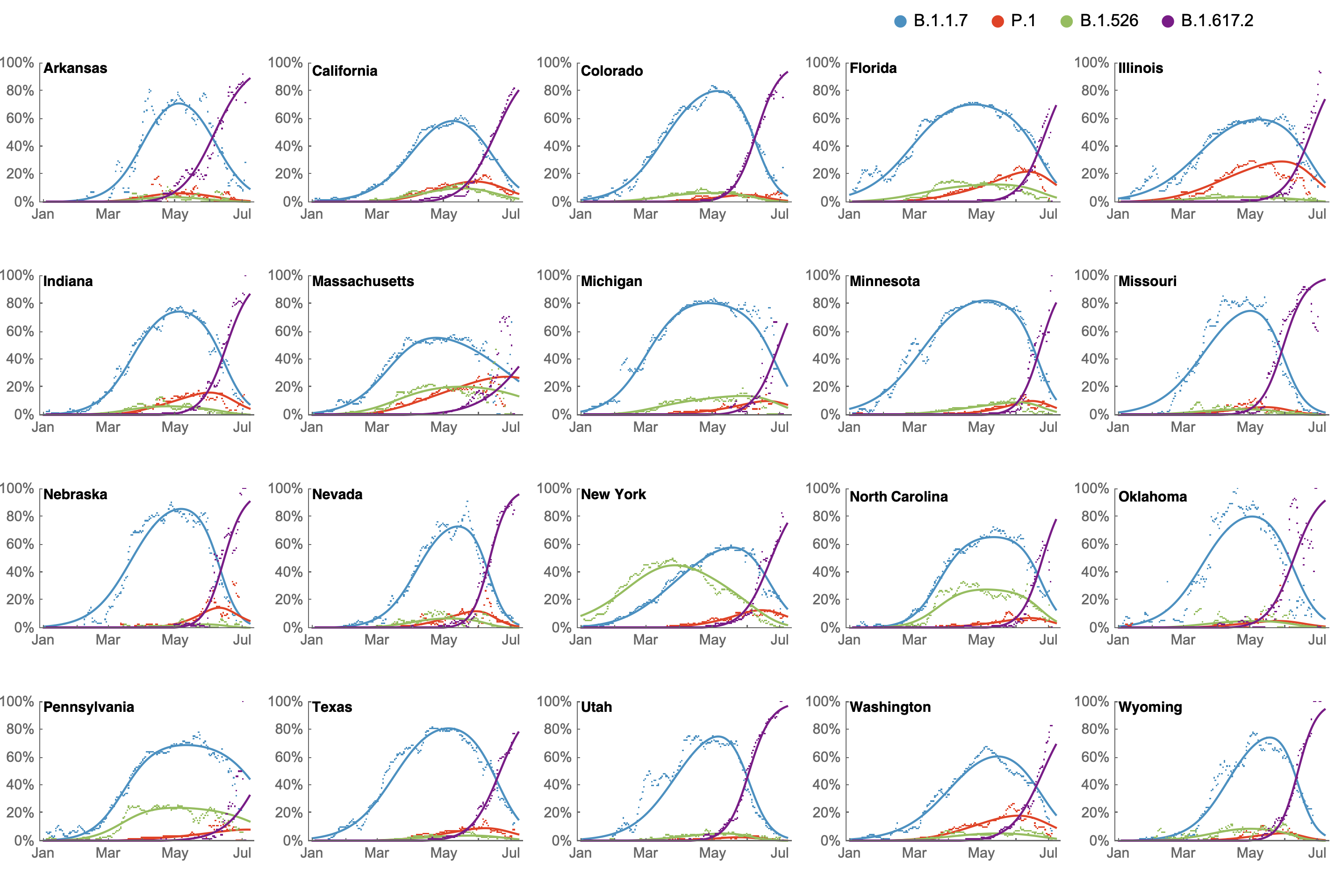## Forecasting

### Forecasting is a big problem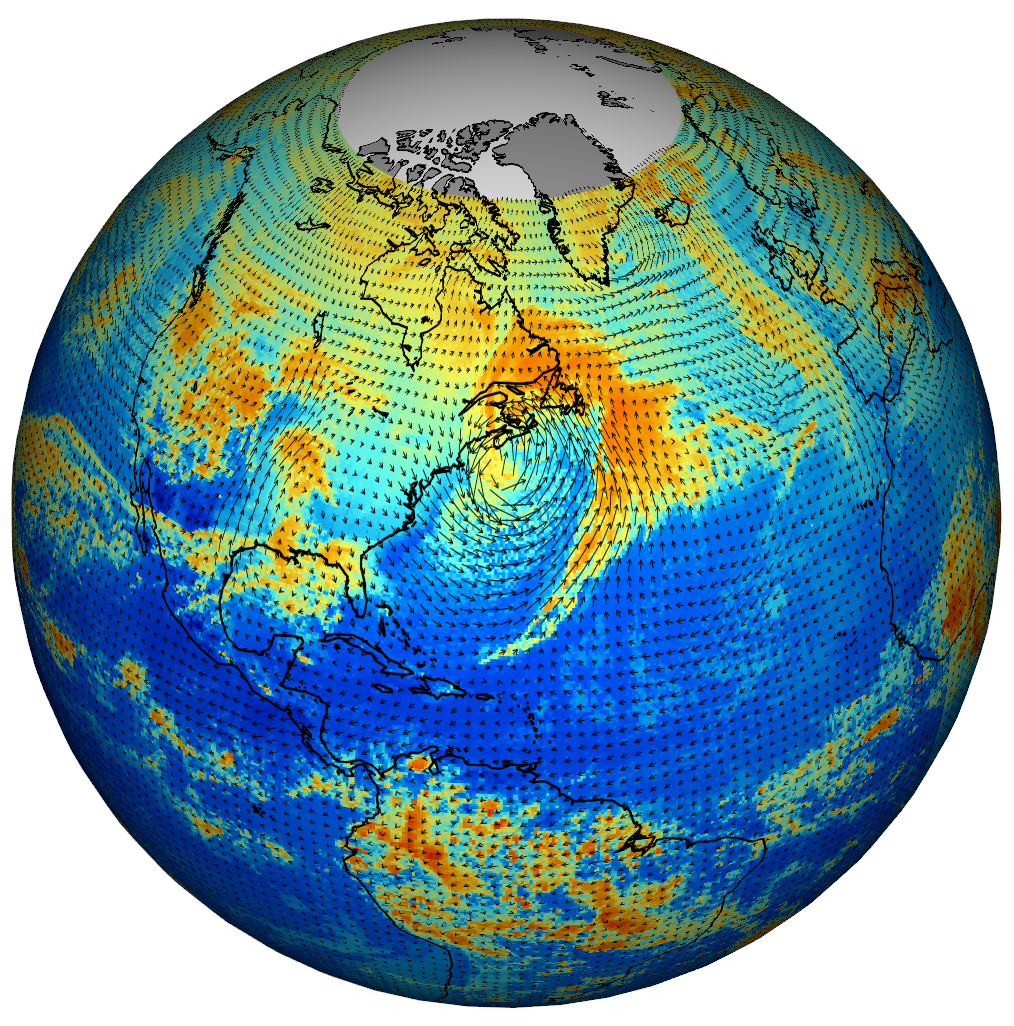### Rapid progress

Actual v. predicted distances of Atlantic cyclones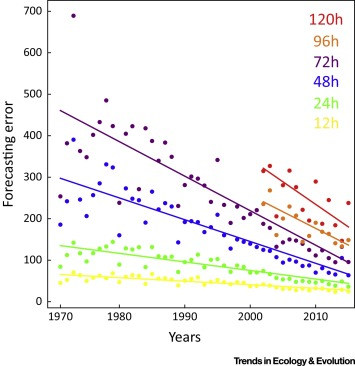### General, overlapping approaches

Machine learning

Statistical models

Nonlinear forecasting

"Mechanistic" modeling

### DREAM challenges

Inference of gene regulatory networks

from knockout, observational, and synthetic data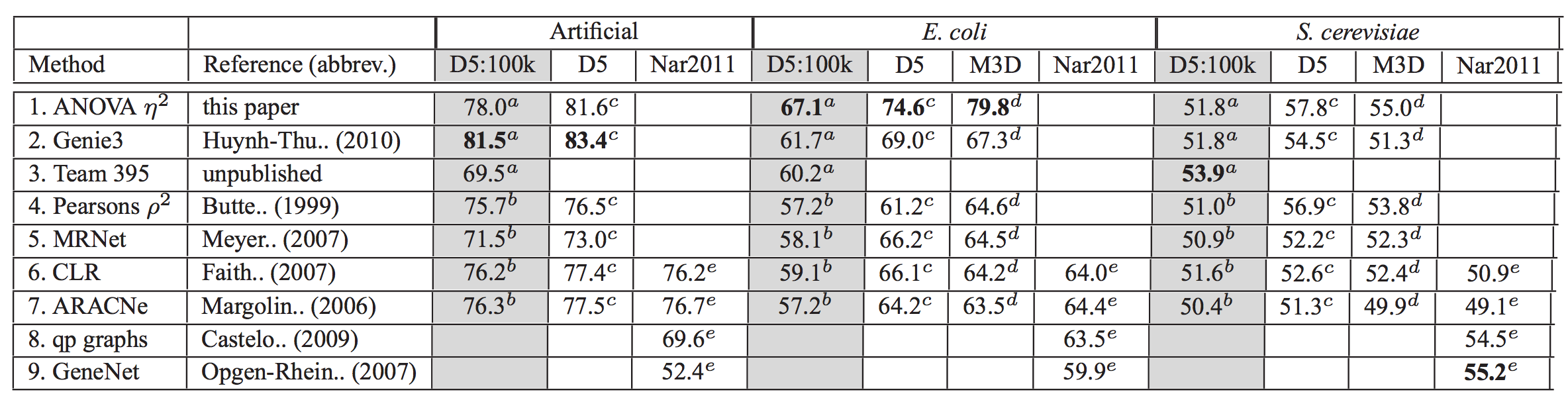Compete Lasso, random forests, Bayesian networks, mutual information, ANOVA, etc.

### Ecological niche modeling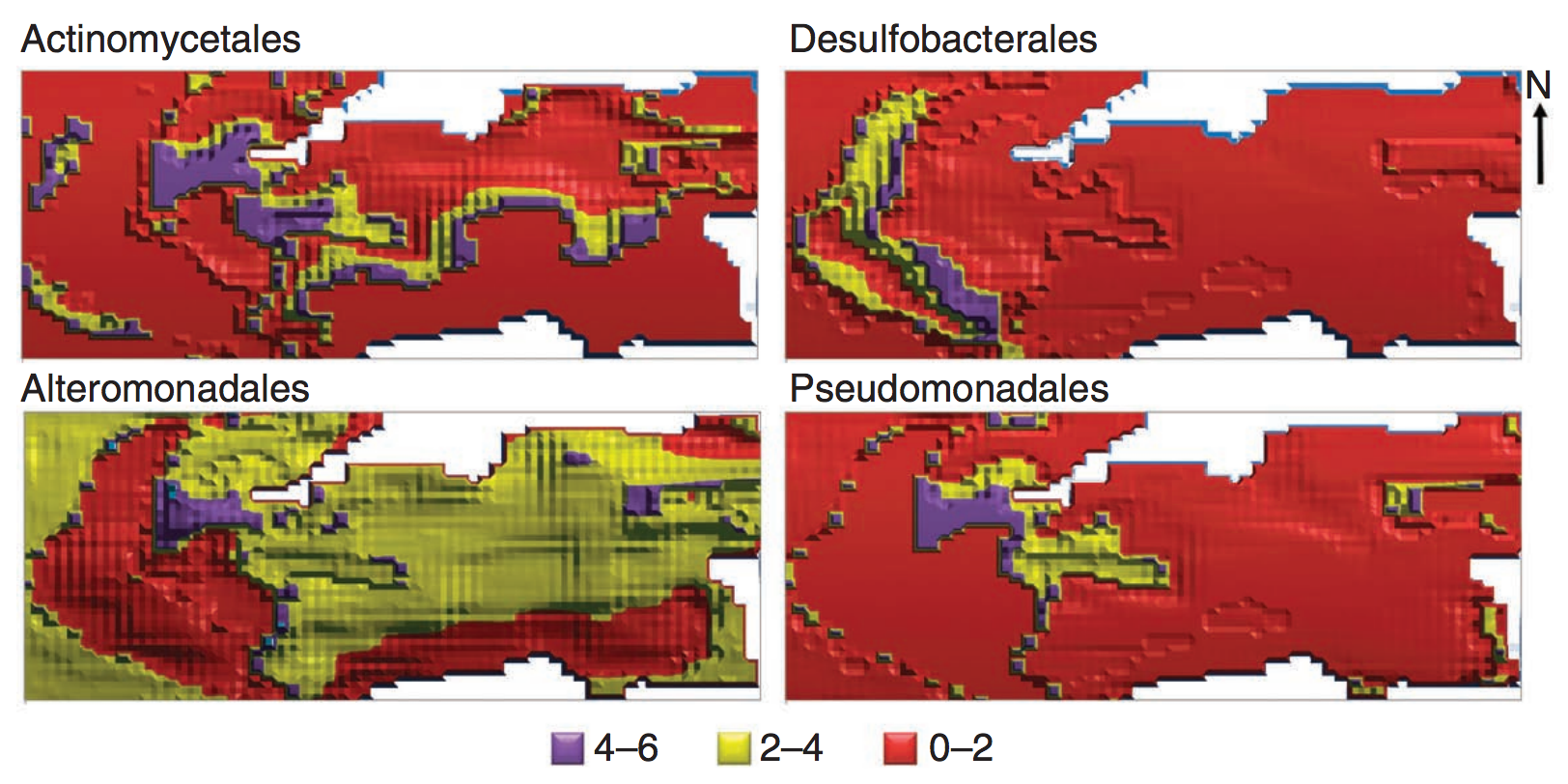An artificial neural network that included microbial interactions performed best.

### Nonlinear forecasting

Reconstruct attractor ("library") from time series

Use attractor to make short-term predictions

### Forecasting communities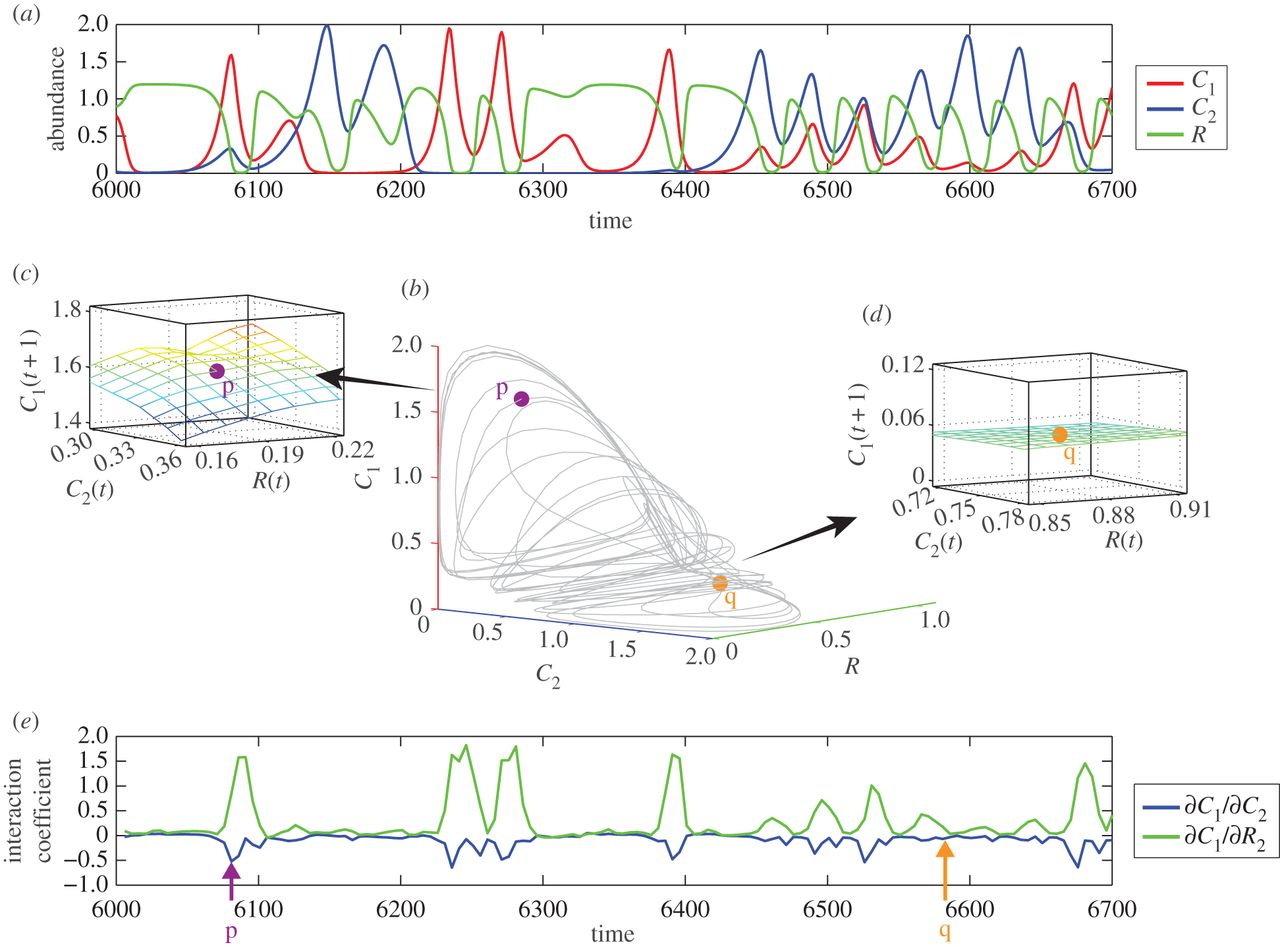### Dynamics may be chaotic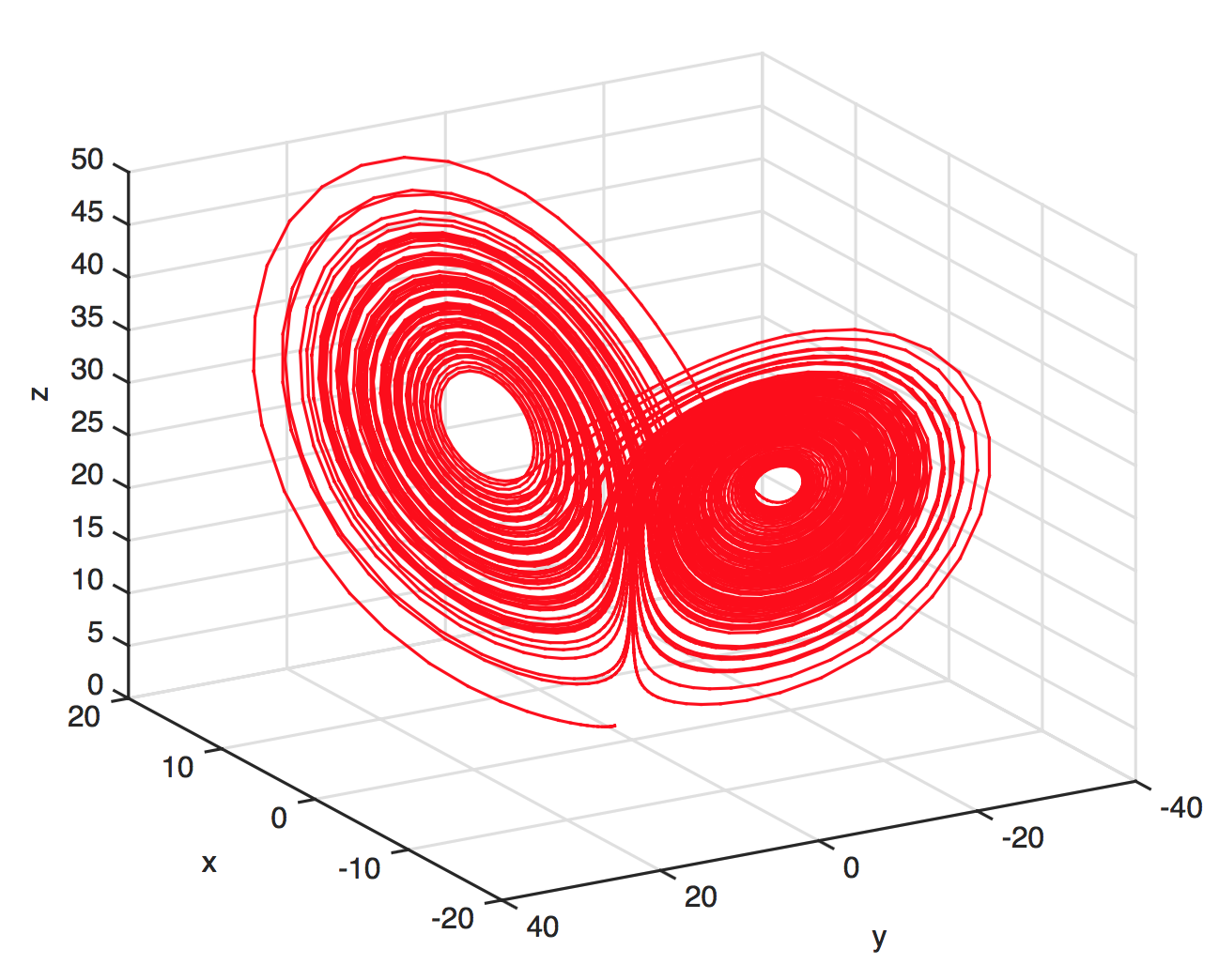### Predictions with chaos: short shelf life

Trajectories in chaotic attractors diverge

$$\lvert \delta \textbf{Z}(t)\rvert \approx e^{\lambda t}\lvert\delta\textbf{Z}_0\rvert$$

$\lambda$ is the Lyapunov exponent

(so with chaos, $\lambda>0$)

### How to forecast

• Choose an embedding dimension $E$ and lag $\tau$
• Each point in $E$-dimensional space: $\{x_t,x_{t-\tau},x_{t-2\tau},...,x_{t-(E-1)\tau}\}$
• Construct these points from the time series
• Define a point to predict ("predictee")
• See where predictee's $E+1$ nearest neighbors wind up $t$ steps into the future
• Measure correlations $\rho$ between predictee's observed future state and neighbors' weighted predictions

### Choosing $E$ and $\tau$

An unsolved problem

Use $E$ (and $\tau$) that yield best predictions### Observational noise v. chaos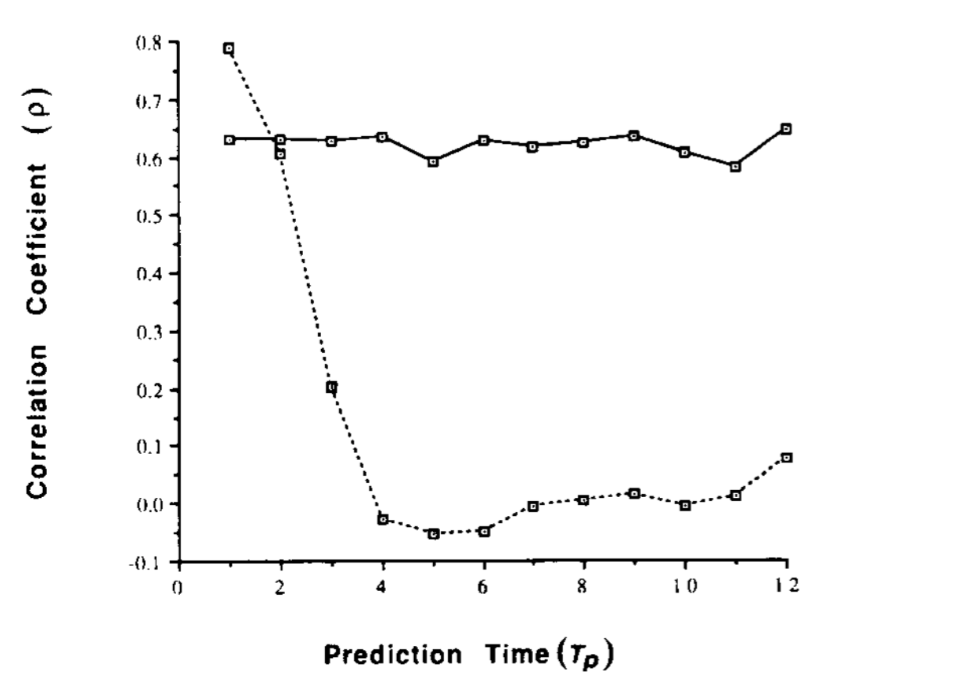### Predicting flu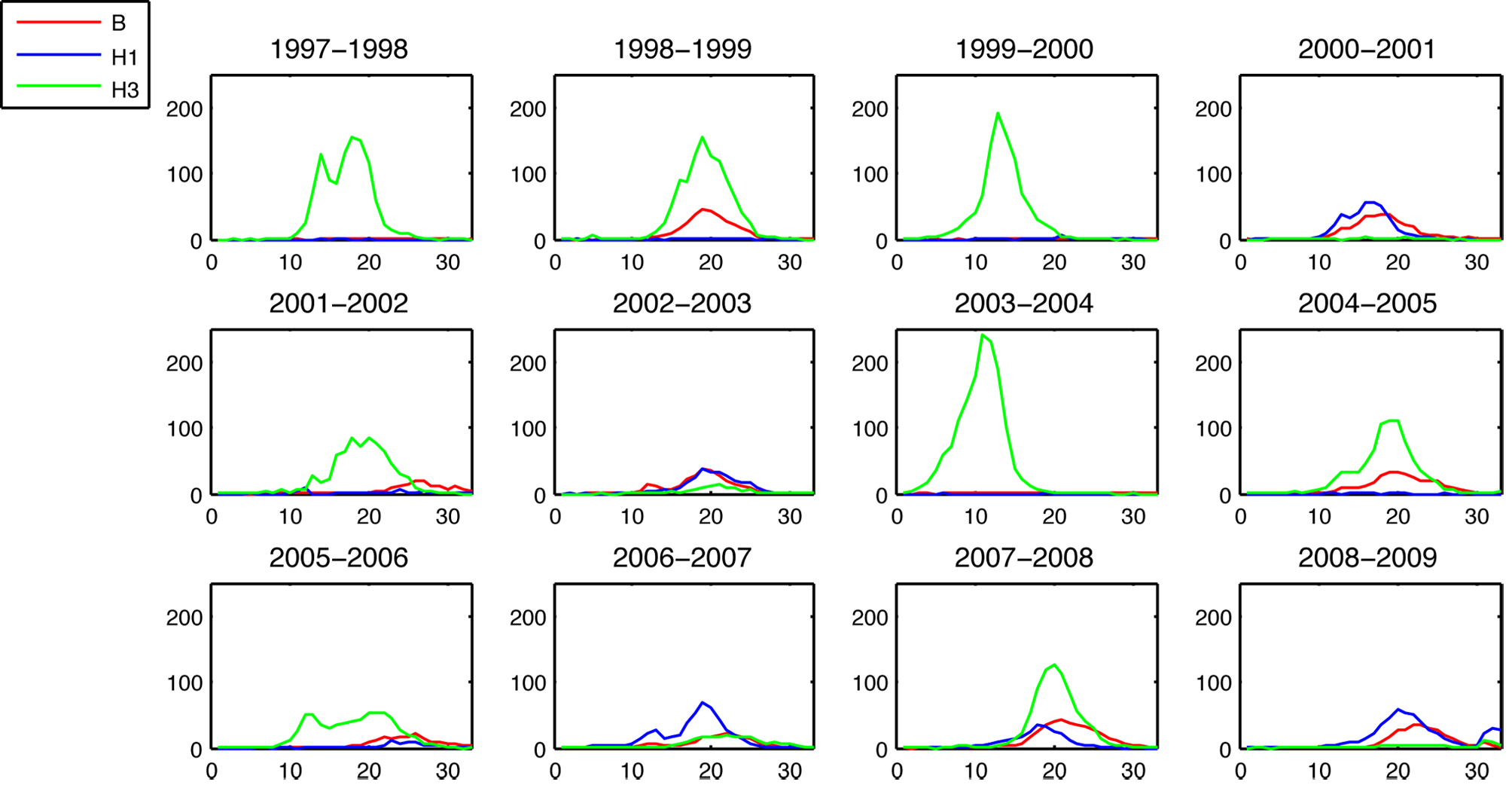### Epidemic sizes negatively correlated### Predict based on cumulative incidence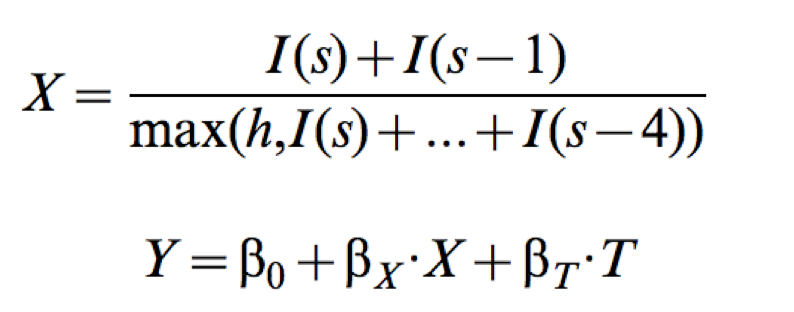where $I(s)$ is the incidence in week $s$, $h$ is a strain-specific incidence threshold, $T$ is the time of crossing $h$, and $Y$ is the strain's whole-season cumulative incidence proxy.

### Predictions v. observations (H3N2)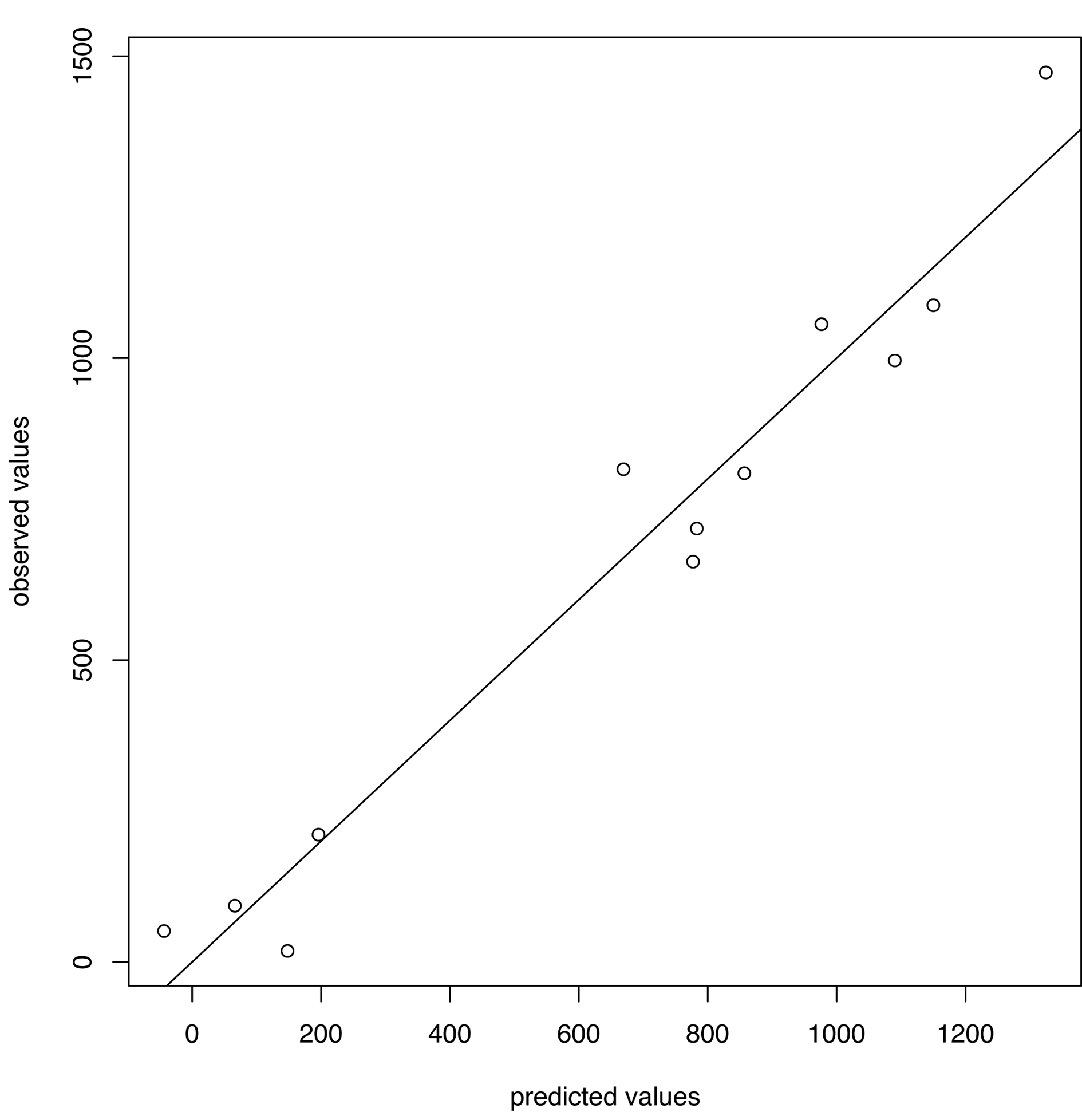### Predicting flu in Hong King

Aim: Predict peak timing and magnitude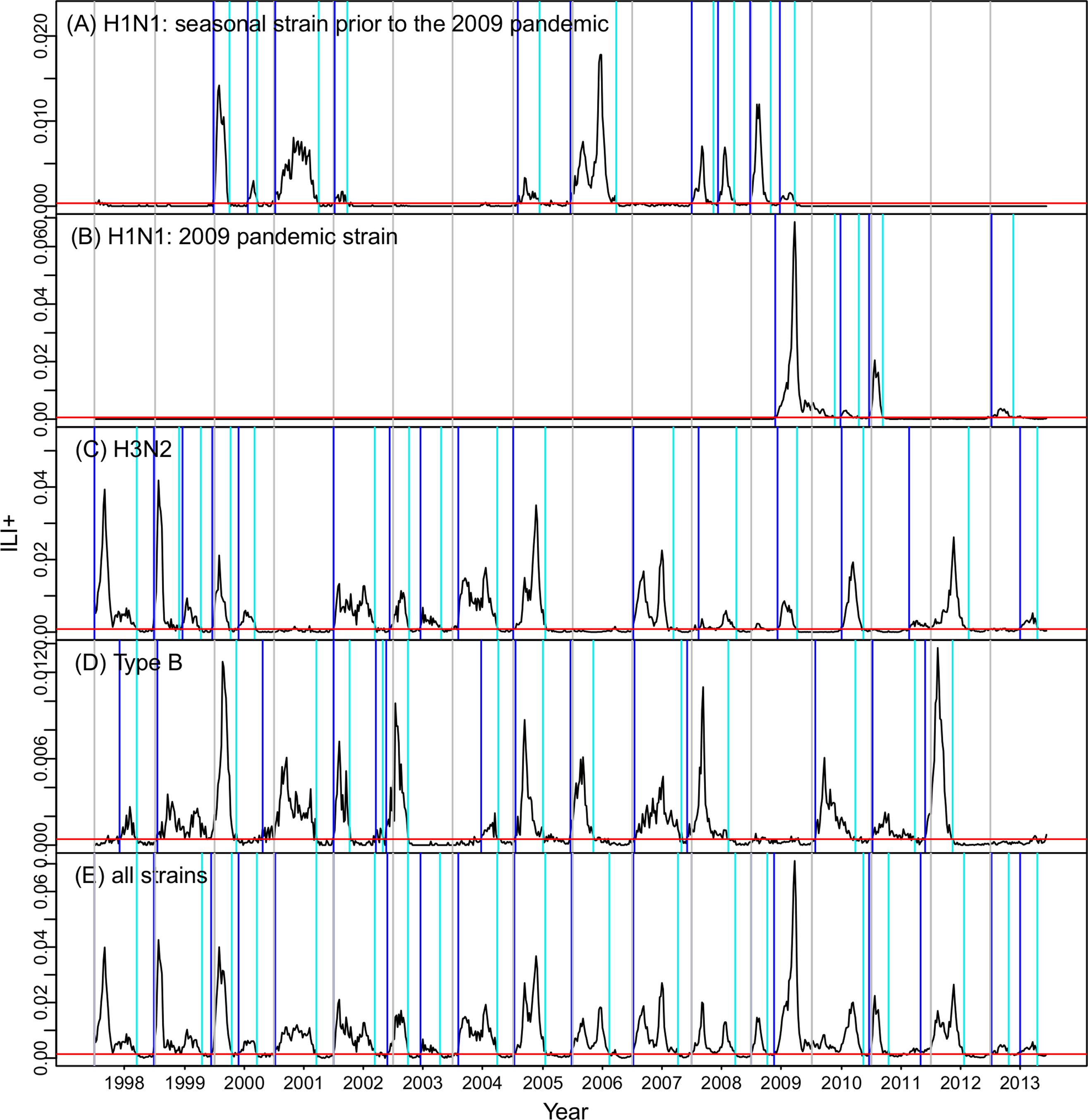### Mechanistic model and particle filterAchieves 37% accuracy with 1-3 week lead, ~50% at 0 week lead

### Forecasting SARS-CoV-2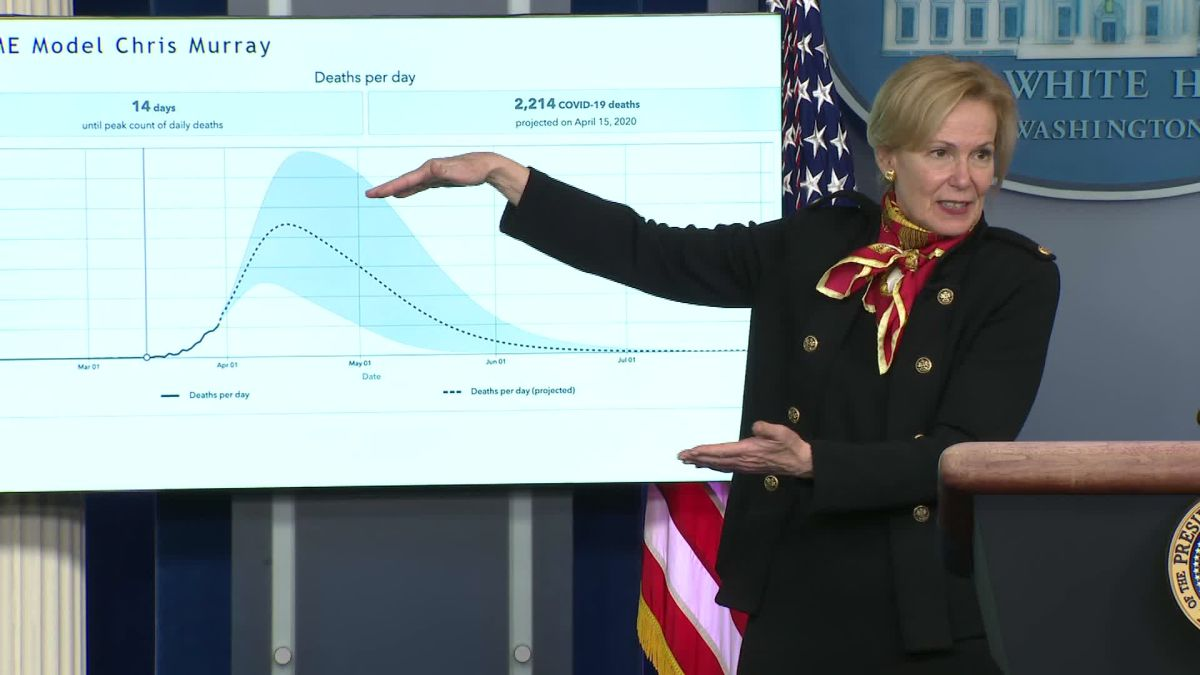### Unusual error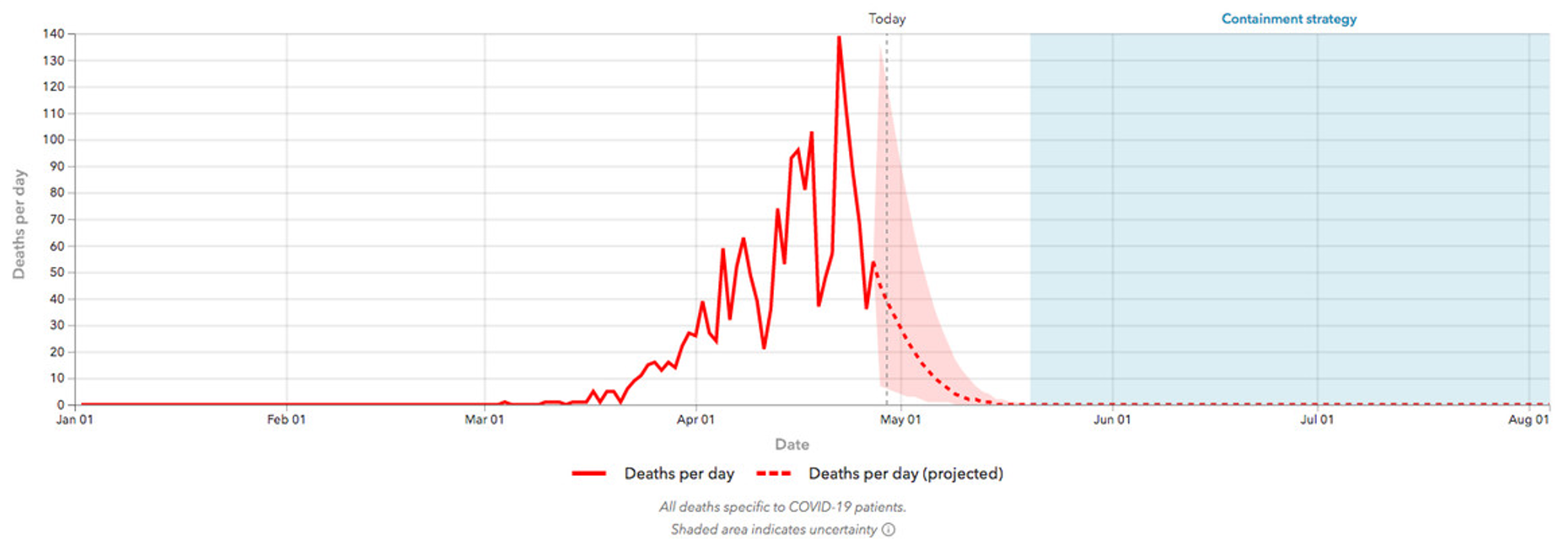### A statistical model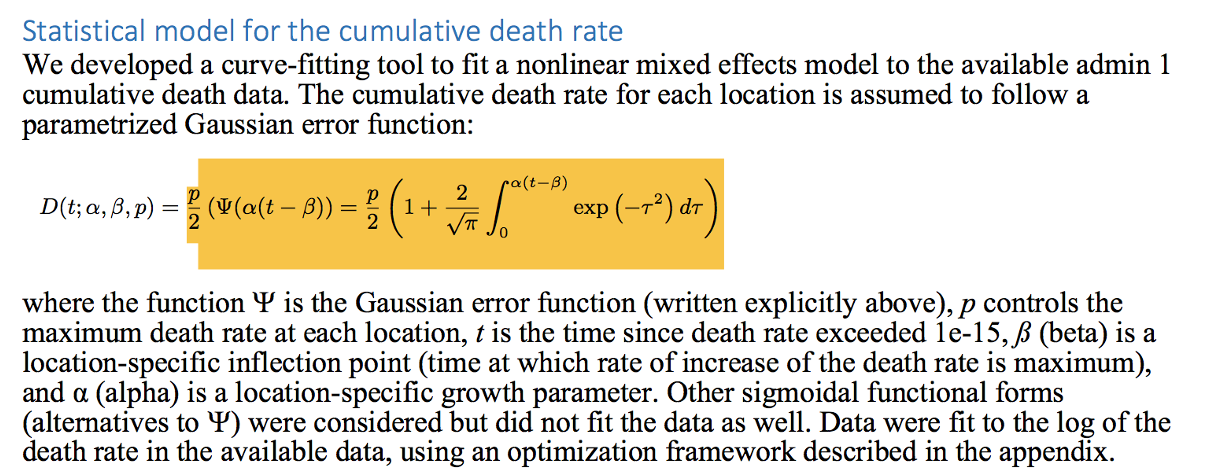### Some precedent: Farr's Law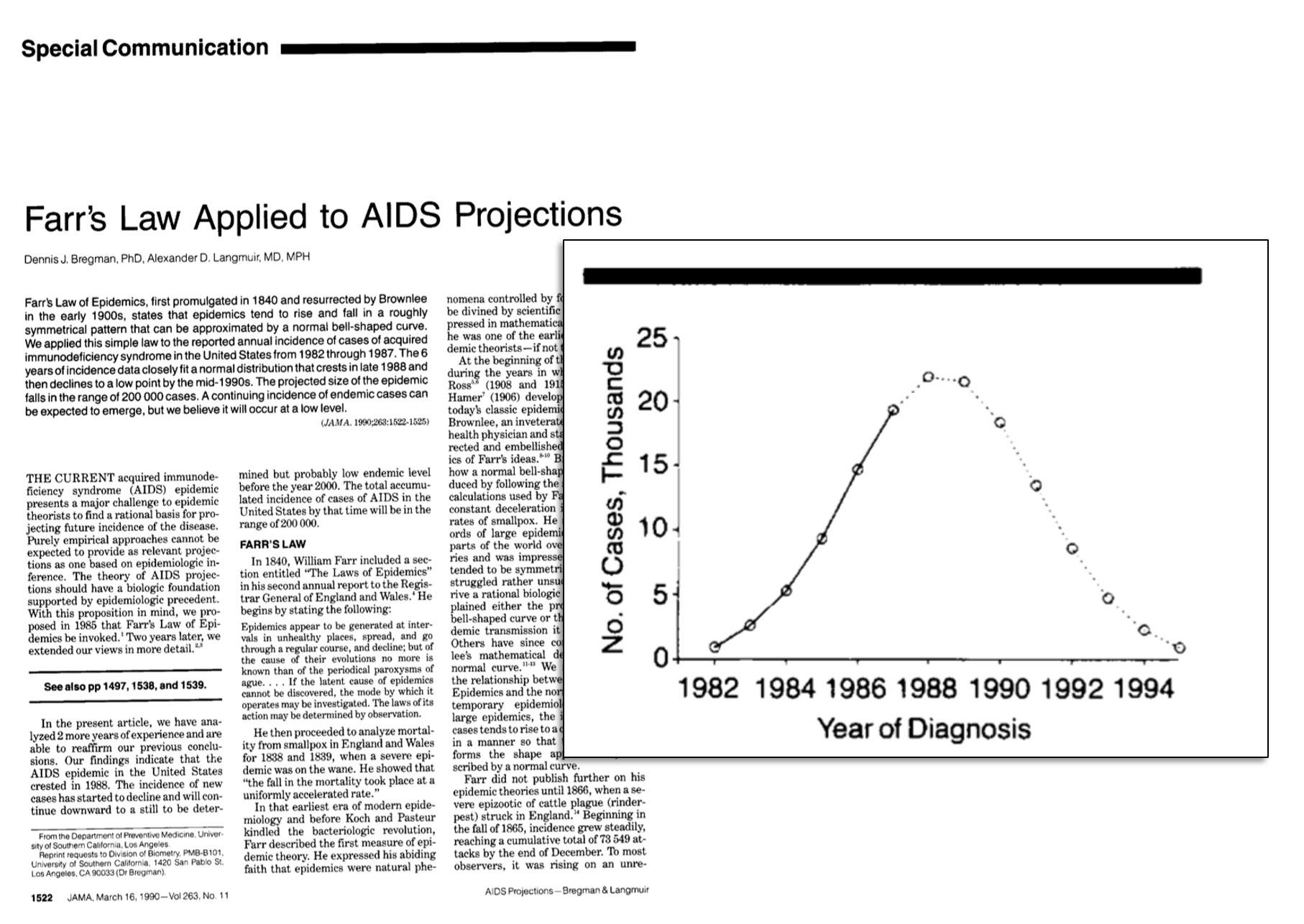### Comparing models### Evaluating performance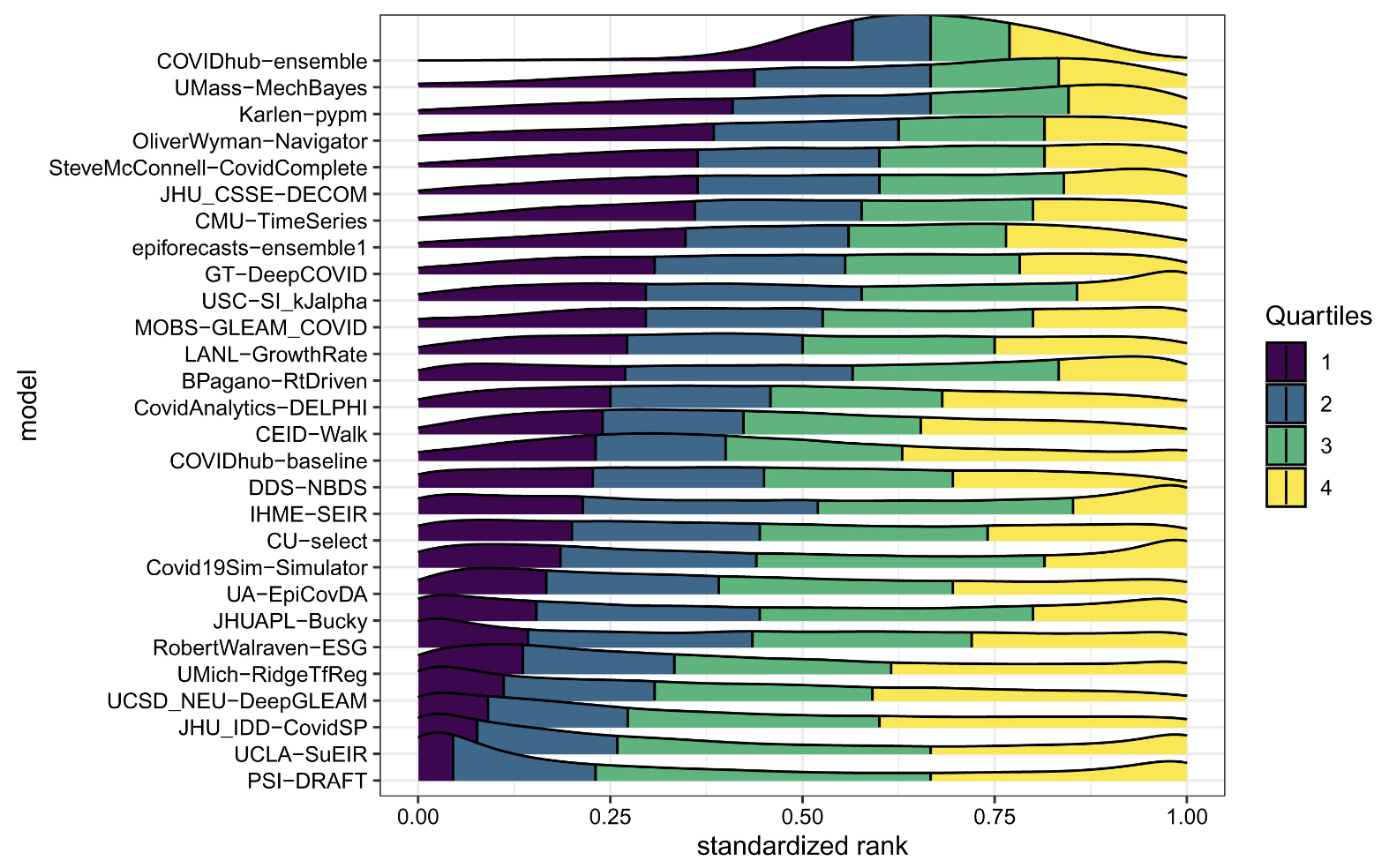### Forecasting flu evolution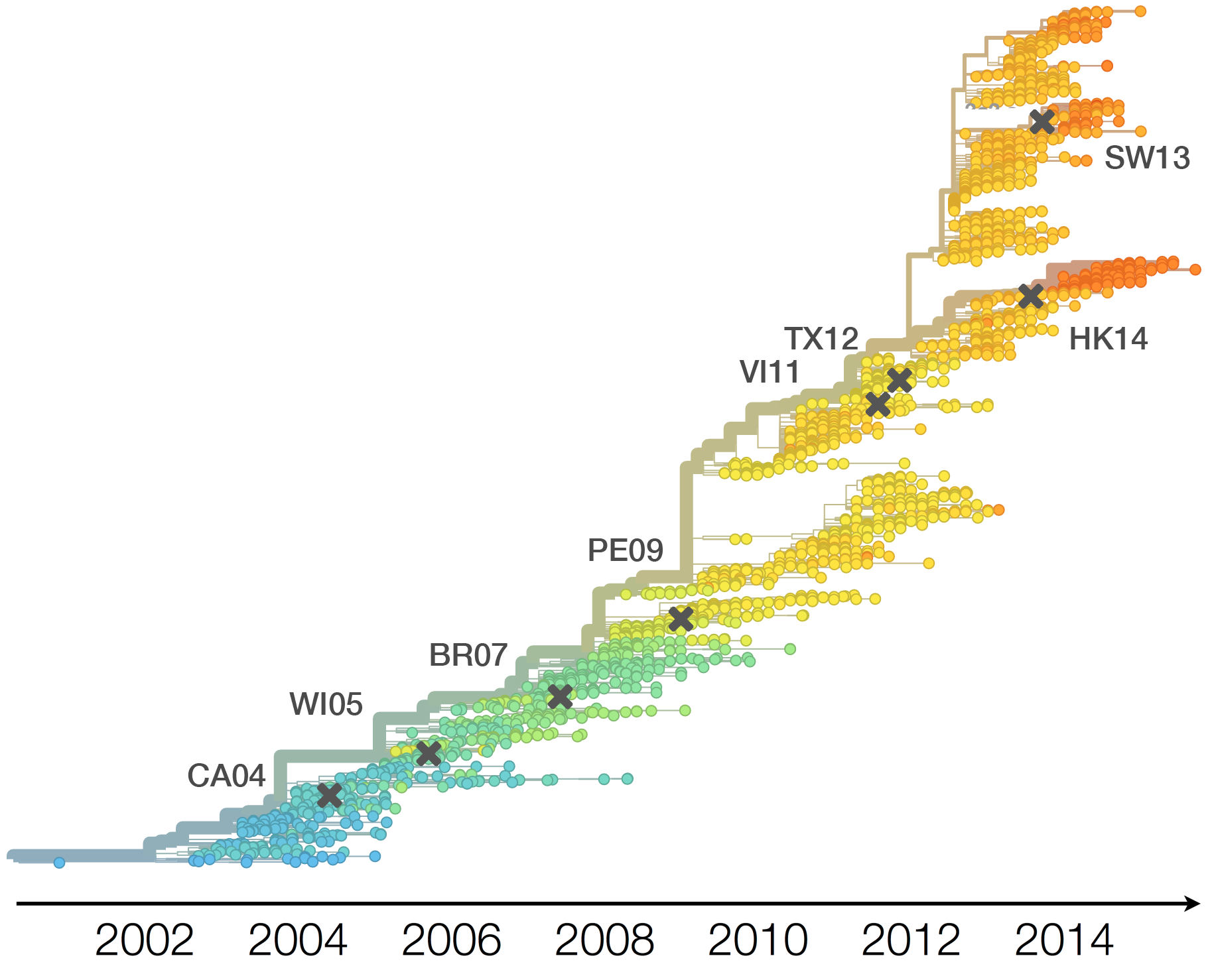### Vaccine strain selection timeline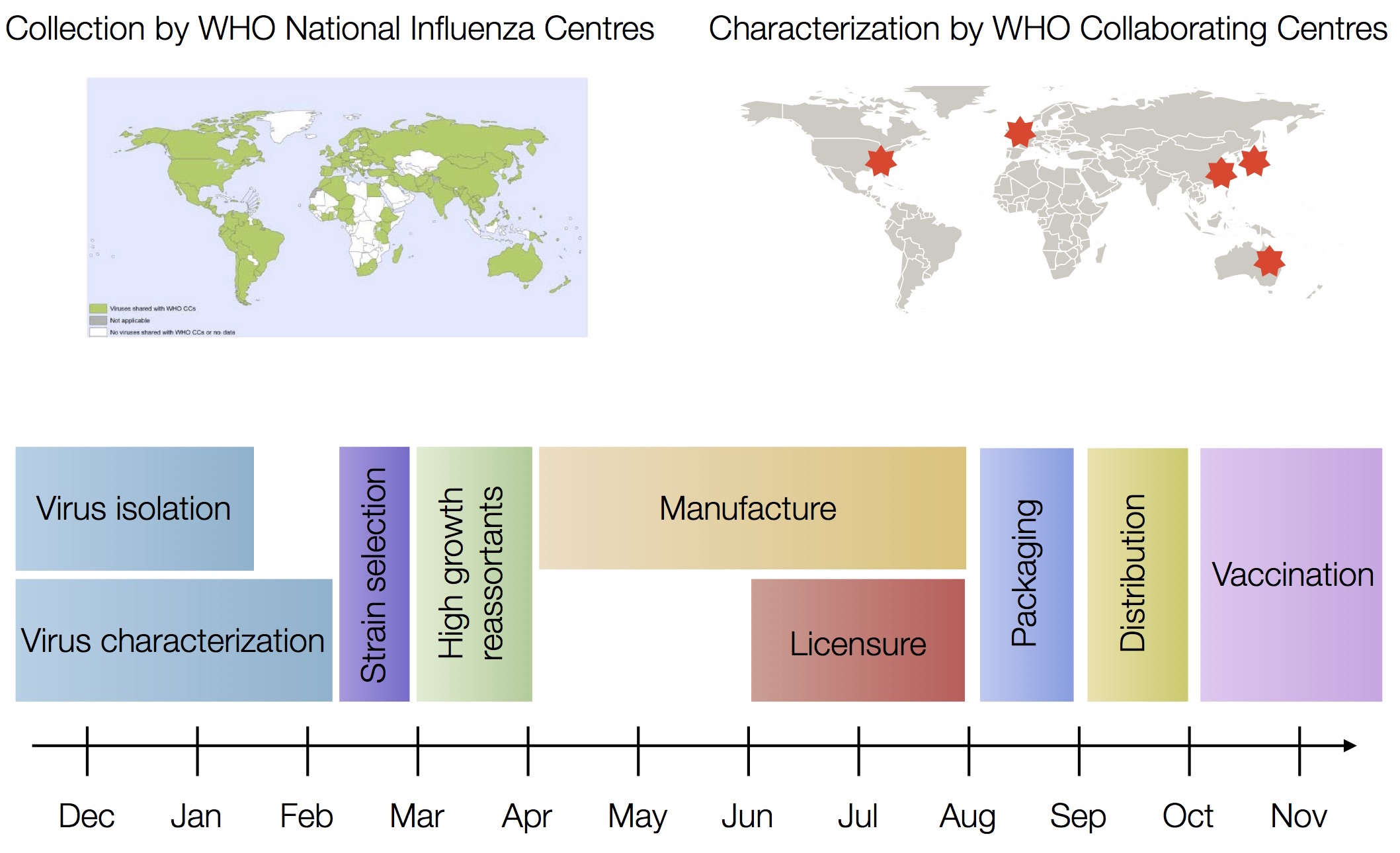### Seek to explain change in clade frequencies over 1 year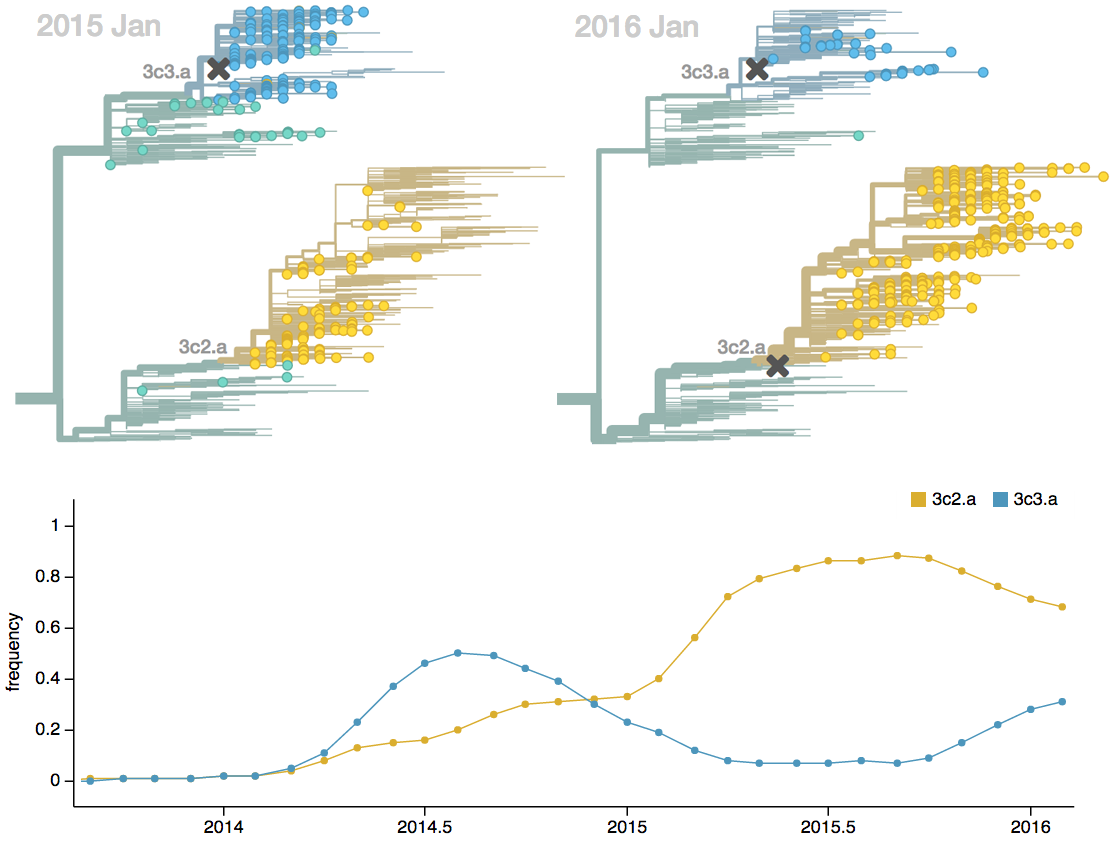### Fitness models can project clade frequencies

Clade frequencies $X$ derive from the fitnesses $f$ and frequencies $x$ of constituent viruses, such that

$$\hat{X}_v(t+\Delta t) = \sum_{i:v} x_i(t) \, \mathrm{exp}(f_i \, \Delta t)$$

This captures clonal interference between competing lineages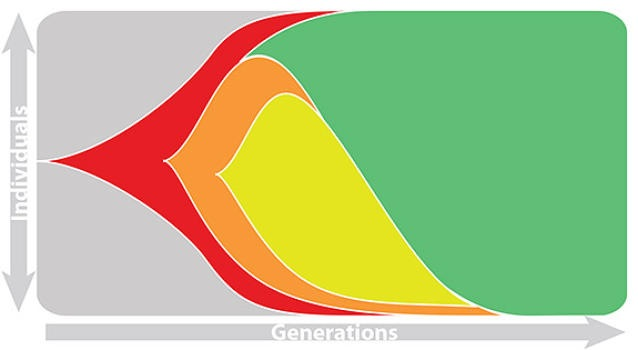### Clonal interference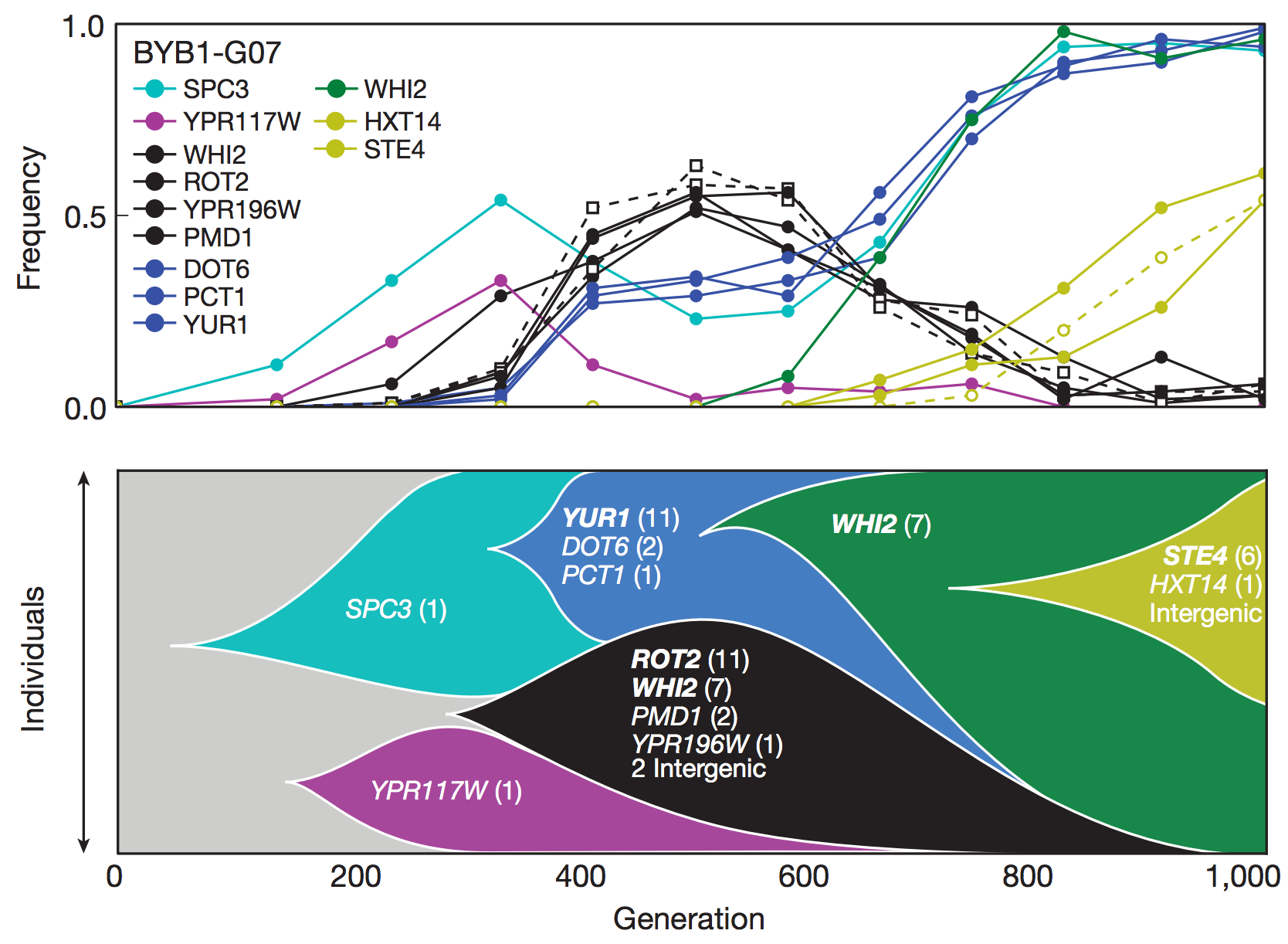### Predictive fitness models

A simple predictive model estimates the fitness $f$ of virus $i$ as

$$\hat{f}_i = \beta^\mathrm{ep} \, f_i^\mathrm{ep} + \beta^\mathrm{ne} \, f_i^\mathrm{ne}$$

where $f_i^\mathrm{ep}$ measures cross-immunity via substitutions at epitope sites and $f_i^\mathrm{ne}$ measures mutational load via substitutions at non-epitope sites

### Model selection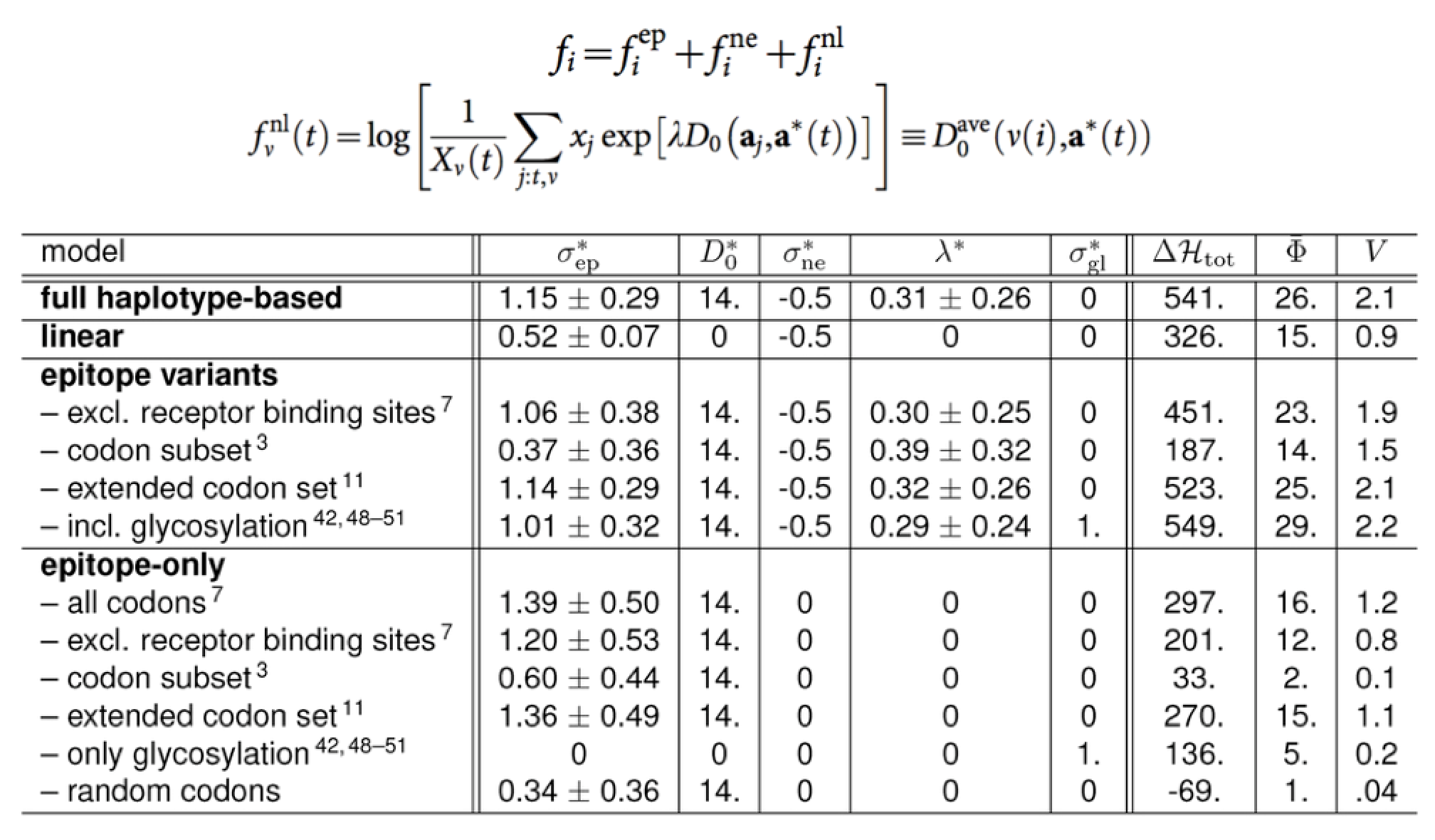### Performance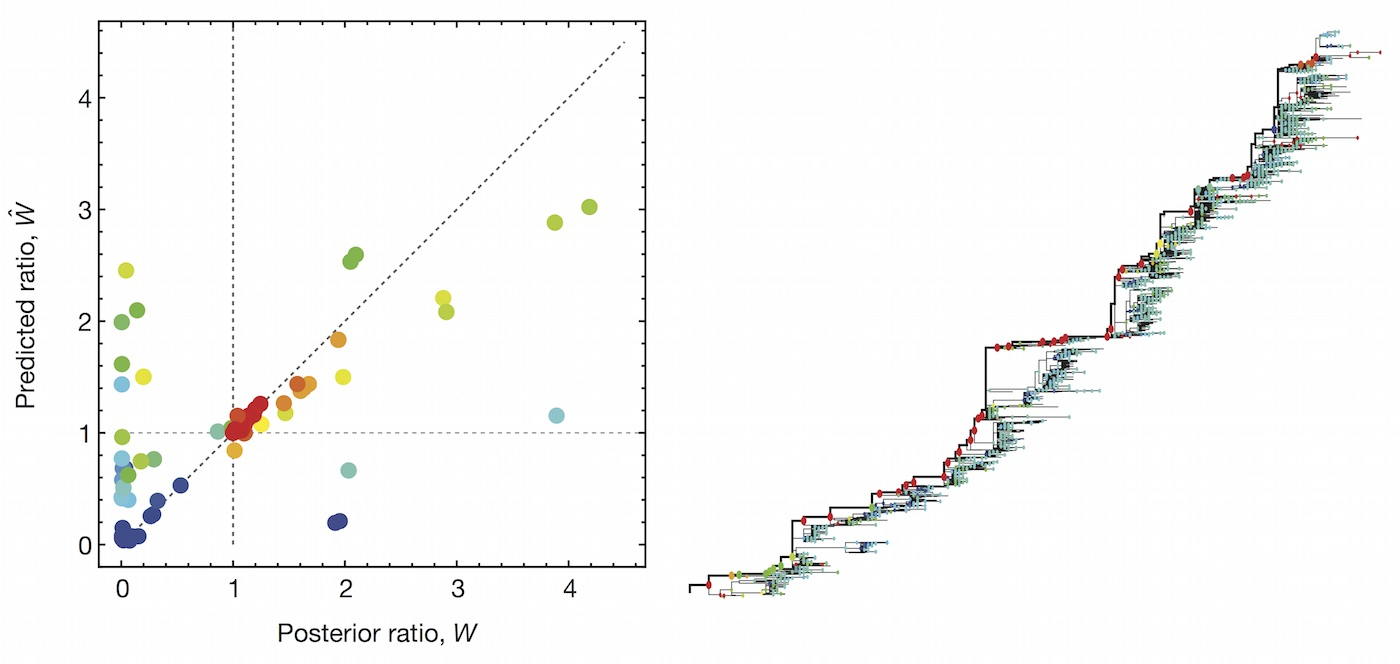### Emergence and spread of SARS-CoV-2 variant viruses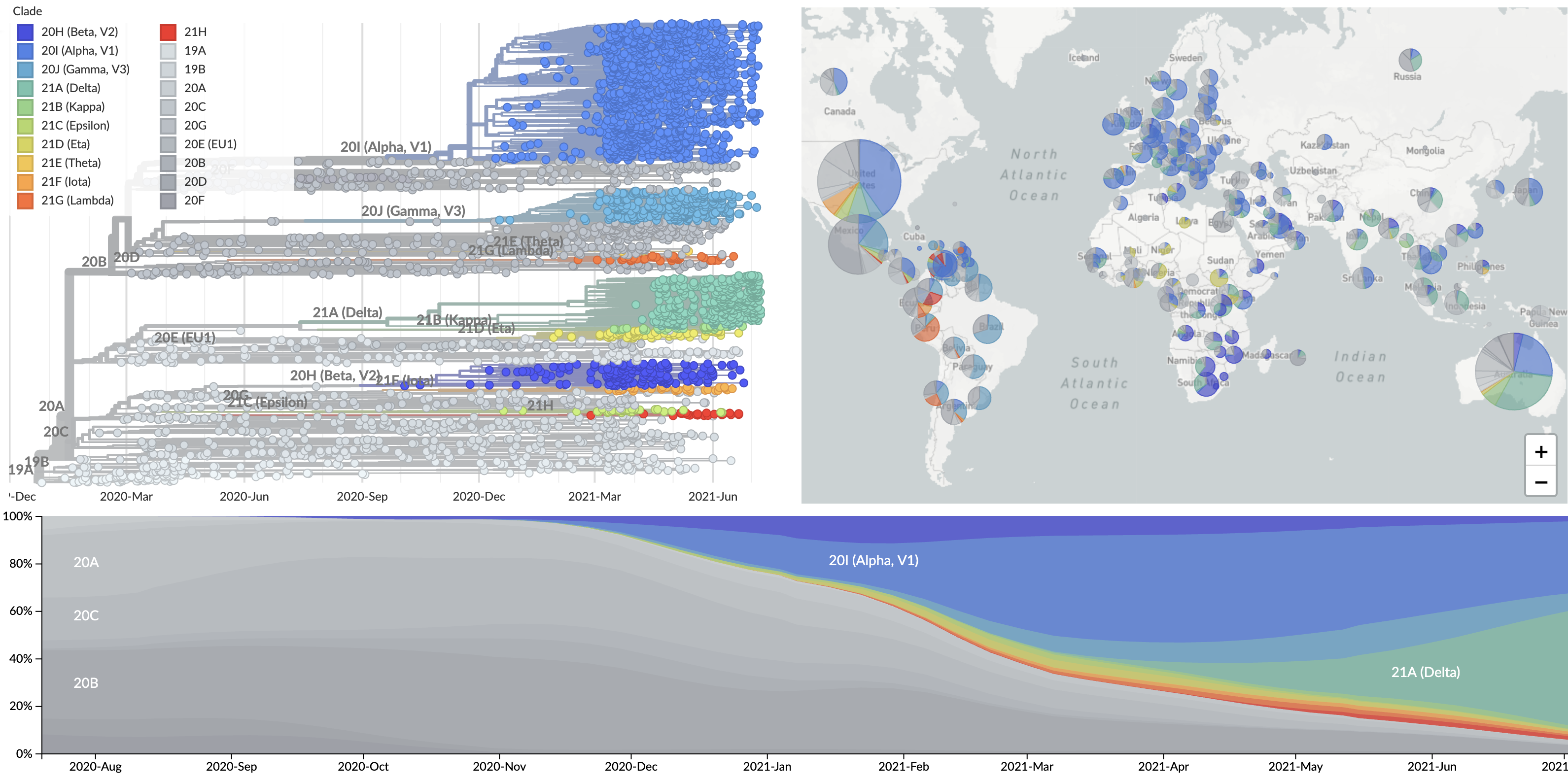### Emergence and spread of SARS-CoV-2 variant viruses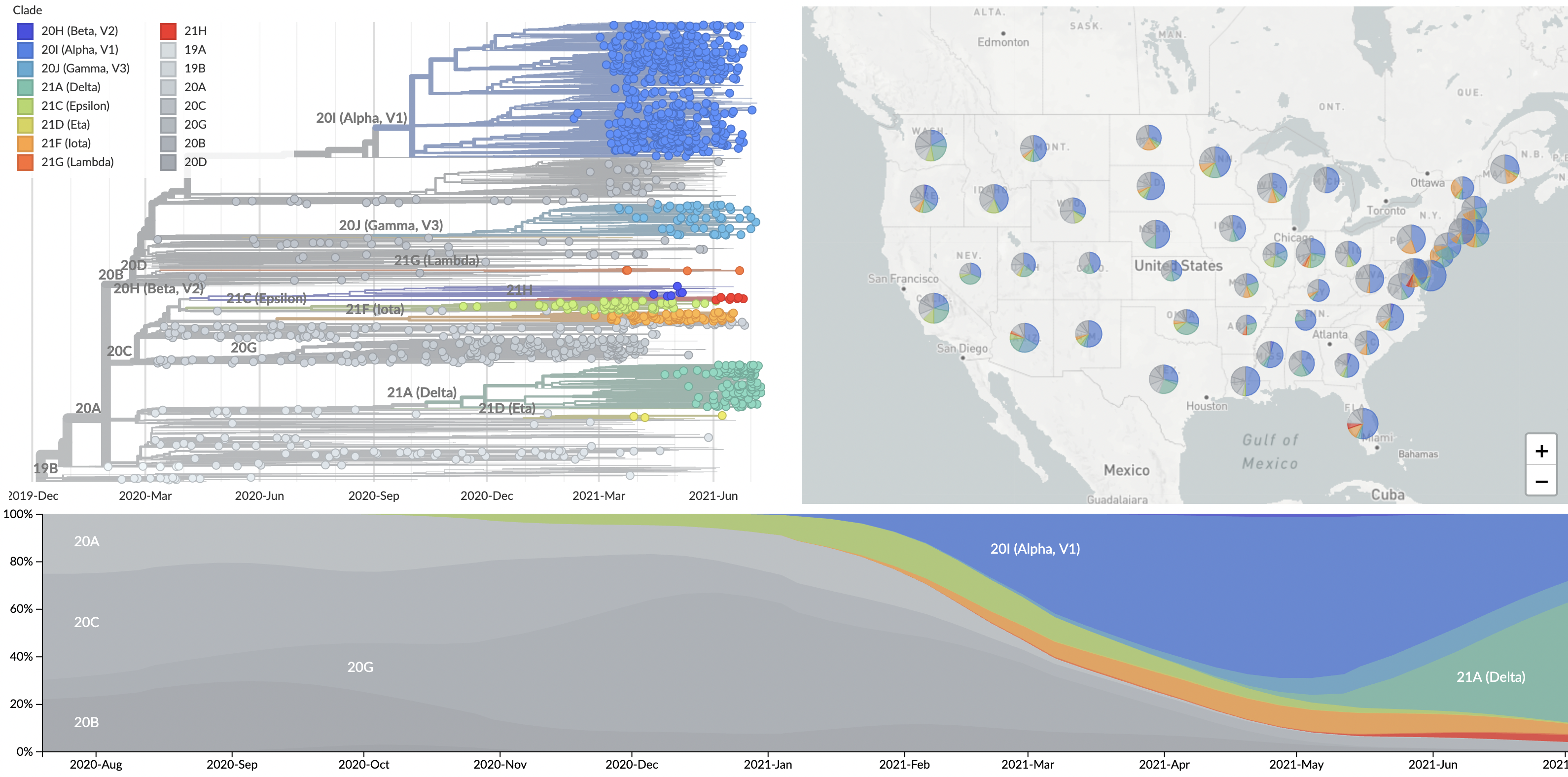### Logistic growth

Exponential growth of fitter variant appears as logistic growth in frequency space

Logistic regression: $$p(x) = \frac{1}{1 + e^{-(\beta_0 + \beta_1 x)}}$$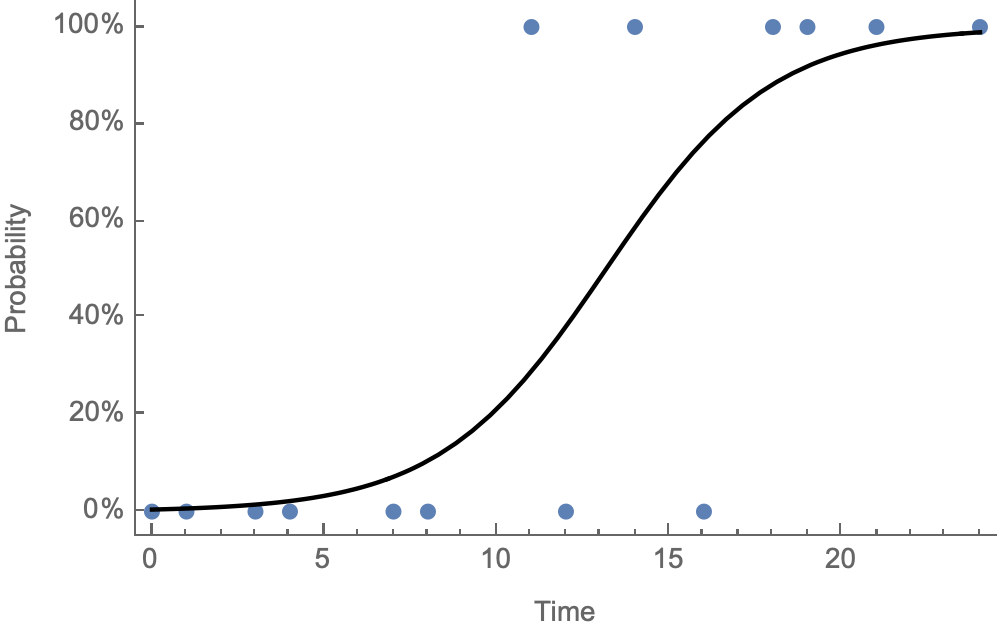### Multinomial logistic regression fit